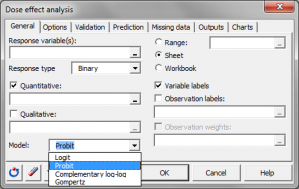# Dose effect analysis

Dose effect analysis is a logistic regression used to model the effect of a dose on a binary outcome. Available in Excel using the XLSTAT software.## What is dose effect analysis

Dose effect analysis is simply a Logistic regression (Logit, Probit, complementary Log-log, Gompertz models) used to model the impact of doses of chemical components (for example a medicine or phytosanitary product) on a binary phenomenon (healing, death).

## Natural mortality in dose effect analysis

Natural mortality should be taken into account in order to model the phenomenon studied more accurately. Indeed, if we consider an experiment carried out on insects, certain will die because of the dose injected, and others from other phenomenon. None of these associated phenomena are relevant to the experiment concerning the effects of the dose but may be taken into account. If p is the probability from a logistic regression model corresponding only to the effect of the dose and if m is natural mortality, then the observed probability that the insect will die is:

P(obs) = m + (1- m) * p

Abbot's formula (Finney, 1971) is written as:

p = (P(obs) – m) / (1 – m)

The natural mortality m may be entered by the user if it is known from previous experiments, or it can be determined by XLSTAT.

## Computing dose effect from from ED01 to ED99 including ED 50, ED 90

XLSTAT calculates ED 50 (or median dose), ED 90 and ED 99 doses which correspond to doses leading to an effect respectively on 50%, 90% and 99% of the population.

## Results for dose effect analysis in XLSTAT

• Goodness of fit coefficients: This table displays a series of statistics for the independent model (corresponding to the case where the linear combination of explanatory variables reduces to a constant) and for the adjusted model.
• Observations: The total number of observations taken into account (sum of the weights of the observations);
• Sum of weights: The total number of observations taken into account (sum of the weights of the observations multiplied by the weights in the regression);
• DF: Degrees of freedom;
• -2 Log(Like.): The logarithm of the likelihood function associated with the model;
• R² (McFadden): Coefficient, like the R2, between 0 and 1 which measures how well the model is adjusted. This coefficient is equal to 1 minus the ratio of the likelihood of the adjusted model to the likelihood of the independent model;
• R²(Cox and Snell): Coefficient, like the R2, between 0 and 1 which measures how well the model is adjusted. This coefficient is equal to 1 minus the ratio of the likelihood of the adjusted model to the likelihood of the independent model raised to the power 2/Sw, where Sw is the sum of weights.
• R²(Nagelkerke): Coefficient, like the R2, between 0 and 1 which measures how well the model is adjusted. This coefficient is equal to ratio of the R² of Cox and Snell, divided by 1 minus the likelihood of the independent model raised to the power 2/Sw;
• AIC: Akaike’s Information Criterion;
• SBC: Schwarz’s Bayesian Criterion.
• Test of the null hypothesis H0: Y=p0: The H0 hypothesis corresponds to the independent model which gives probability p0 whatever the values of the explanatory variables. We seek to check if the adjusted model is significantly more powerful than this model. Three tests are available: the likelihood ratio test (-2 Log(Like.)), the Score test and the Wald test. The three statistics follow a Chi2 distribution whose degrees of freedom are shown.
• Type III analysis: This table is only useful if there is more than one explanatory variable. Here, the adjusted model is tested against a test model where the variable in the row of the table in question has been removed. If the probability Pr > LR is less than a significance threshold which has been set (typically 0.05), then the contribution of the variable to the adjustment of the model is significant. Otherwise, it can be removed from the model.
• Model parameters: The parameter estimate, corresponding standard deviation, Wald's Chi2, the corresponding p-value and the confidence interval are displayed for the constant and each variable of the model. If the corresponding option has been activated, the "profile likelihood" intervals are also displayed.
• Model equation: The equation of the model is then displayed to make it easier to read or re-use the model.
• Standardized coefficients table: The table of standardized coefficients (also called beta coefficients) is used to compare the relative weights of the variables. The higher the absolute value of a coefficient, the more important the weight of the corresponding variable. When the confidence interval around standardized coefficients has value 0 (this can be easily seen on the chart of normalized coefficients), the weight of a variable in the model is not significant.
• Predictions and residuals table: The predictions and residuals table shows, for each observation, its weight, the value of the qualitative explanatory variable, if there is only one, the observed value of the dependent variable, the model's prediction, the same values divided by the weights, the standardized residuals and a confidence interval.
• Probability analysis table: If only one quantitative variable has been selected, the probability analysis table allows to see to which value of the explanatory variable corresponds a given probability of success.### analyze your data with xlstat

14-day free trial

Included in